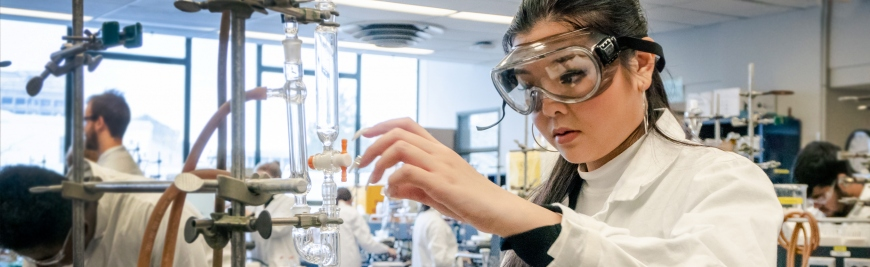## Research & Teaching Faculty# The quadrature discretization method (QDM) in the calculation of the rotational-vibrational transitions in rare gas dimers

 Title The quadrature discretization method (QDM) in the calculation of the rotational-vibrational transitions in rare gas dimers Publication Type Journal Article Year of Publication 1997 Authors Shizgal, BD Journal Theochem-Journal of Molecular Structure Volume 391 Pagination 131-139 Date Published Feb Type of Article Article ISBN Number 0166-1280 Keywords AR-XE, COEFFICIENTS, DISCRETE-VARIABLE REPRESENTATION, NE-KR, ORDINATE METHOD, POTENTIALS, quadrature discretion method, QUANTUM-MECHANICS, rare gas dimer, rotation-vibration, SCHRODINGER-EQUATION, Schroedinger equation, SPECTRA, STATES, TRANSITION Abstract A discretization method referred to as the Quadrature Discretization Method (QDM) is employed for the determination of the rotational-vibrational states of the inert gas dimers. The QDM is based on the discretization of the wave function on a grid of points that coincide with the points of a quadrature. The quadrature is based on a set of non-classical polynomials orthogonal with respect to a weight function determined by the potential function in the Schroedinger equation. In this paper, the weight functions used in the solution of the Schroedinger equation are related to the ground state wavefunctions of the Morse potential that approximates the potential of interest. Calculations are carried out for vibrational energy levels for HF modelled with a Morse potential. Excellent agreement is obtained with the known spectrum of the Morse potential. The rate of convergence of the eigenvalues and the eigenfunctions of the Schroedinger equation is very rapid with this approach. The vibrational and rotational transitions for the rare gas dimers are calculated and compared with the results reported recently by Ogilvie and Wang (J. Mol. Struc., 291 (1993) 313). (C) 1997 Elsevier Science B.V. URL ://A1997WU75700014